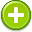#gradient 中文解釋 wordnet sense Collocation Usage
Noun
/ˈgrādēənt/,Font size:1. An inclined part of a road or railway; a slope
• - fail-safe brakes for use on steep gradients
2. The degree of such a slope
• - the path becomes very rough as the gradient increases
3. The degree of steepness of a graph at any point

4. An increase or decrease in the magnitude of a property (e.g., temperature, pressure, or concentration) observed in passing from one point or moment to another

5. The rate of such a change

6. The vector formed by the operator ∇ acting on a scalar function at a given point in a scalar field

1. a graded change in the magnitude of some physical quantity or dimension
2. the property possessed by a line or surface that departs from the horizontal; "a five-degree gradient"
3. In vector calculus, the gradient of a scalar field is a vector field which points in the direction of the greatest rate of increase of the scalar field, and whose magnitude is the greatest rate of change.
4. Gradience as a linguistic concept was first advanced by linguist Dwight Bolinger to describe the effect seen in the spectrum of probability. Gradience, as word or trope, is visually represented with signs or images.
5. A slope or incline; A rate of inclination or declination of a slope; Of a function y = f(x) or the graph of such a function, the rate of change of y with respect to x, that is, the amount by which y changes for a certain (often unit) change in x; The rate at which a physical quantity increases ...
6. (Gradients) Areas of land that slope downwards.
7. (Gradients) are often oversimplified. Dysesthesia follows loss of fine touch, while hyperpathia follows retention of fine touch. By convention, however, these usually occur distally and proximally respectively, as described below.
8. The difference in pressure, oxygen tension, or other variable as a function of distance, time or other continuously changing influence.
9. a gradual approach to something, taken step by step, so that, finally, quite complicated and difficult activities or concepts can be achieved with relative ease.
10. (1) A change of elevation, velocity, pressure, or other characteristics per unit length. (2) Slope.
11. Spring gradient, or change in load per unit of deflection.
12. The rate of change (first derivatives of the energy of a molecular system as a function of atomic positions. A gradient of zero indicates a configuration with a minimum energy.
13. A transition of color, creating a blended change between screen percentages of a single color or between two different colors.
14. Amount by which the grade increases or decreases in a unit of horizontal distance (21).
15. (gra'-di-ent) Applied to a tortoise represented as walking.
16. If f is a function of two variables, then the gradient of f at a given point is the vector sum of the partial derivative of f with respect to the first variable times the vector i, plus the partial derivative of f with respect to the second variable times the vector j. ...
17. A rate of change with respect to distance of a variable quantity, as temperature or pressure, in the direction of maximum change.
18. A gradient is a gradual transition of colors. Many metallic images are gradients. Web images that use gradient fills as a special effect should be saved in a JPEG rather than a GIF format.
19. The time rate or spatial rate of change of an atmospheric property.
20. A variation in some quantity with respect to another. In the context of NMR, a magnetic field gradient is a variation in the magnetic field with respect to distance. [Chapter 7]
21. The amount of vertical drop a stream experiences over a given distance.
22. General slope or rate of change in vertical elevation per unit of horizontal distance of water surface of a flowing stream. Slope along a specific route, as of a road surface, channel or pipe.
23. Change rate of any element value with distance in any given direction.
24. A vector of partial derivatives of a function that operates on vectors. Intuitively, the gradient represents the slope of a high-dimensional surface.
25. [JP 1-02] (DoD) The rate of inclination to horizontal expressed as a ratio, such as 1:25, indicating a one unit rise to 25 units of horizontal distance.